# Find Current Ix Using Superposition Theorem - Basic Electrical and Electronics Engineering

Sum

Find current IX using Superposition theorem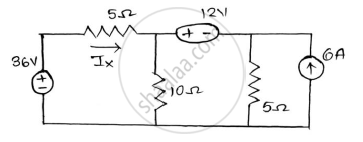#### Solution

(1) 36V is active other all are inactive.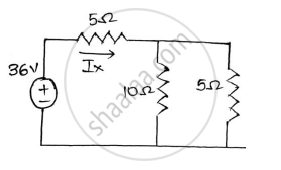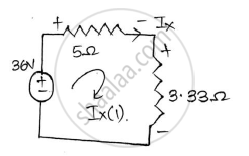10Ω || 5Ω = 3.33Ω
Applying KCL to the circuit.
−36𝑉+5𝐼𝑋+3.33𝐼𝑋=0
8.33𝐼𝑋=36
𝐼𝑋=4.321Am

(2) 12V is active other all are inactive.
Applying KVL at mesh 1
−5𝐼1+10(𝐼1−𝐼2)=0
−5𝐼1+10𝐼1−10𝐼2=0 ……..(1)
Applying KVL at mesh 2
12−5𝐼2−10(𝐼2−𝐼1)=0

12=−10𝐼1+15𝐼2 ………..(2)
From (1) and (2) we get,
𝐼1=2.142𝐴 and 𝐼2=1.0714𝐴
𝐼𝑋(2)=2.142 Am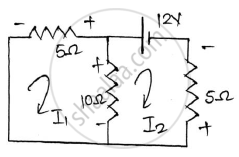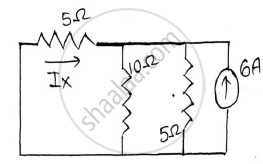(3) 6A is active and other all are inactive.
10Ω || 5Ω = 3.33Ω

𝐼𝑋(3)=6 x 3.33/(3.33+5)= 2.398 Am

𝐼𝑋(3)=−2.398 Am

𝐼𝑋= −2.398+2.142+4.32=4.065

𝑰𝑿=𝟒.𝟎𝟔𝟓 Am

Concept: Superposition Theorem
Is there an error in this question or solution?